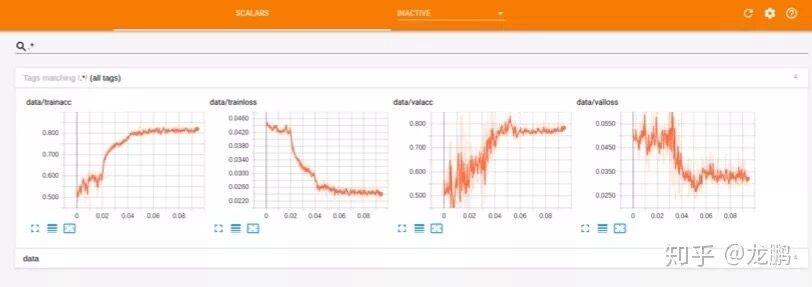# 【pytorch速成】Pytorch图像分类从模型自定义到测试

【pytorch速成】Pytorch图像分类从模型自定义到测试

【caffe速成】caffe图像分类从模型自定义到测试

【tensorflow速成】Tensorflow图像分类从模型自定义到测试

01 什么是 Pytorch

Torch 是纽约大学的一个机器学习开源框架，几年前在学术界非常流行，包括 Lecun等大佬都在使用。但是由于使用的是一种绝大部分人绝对没有听过的 Lua 语言，导致很多人都被吓退。后来随着 Python 的生态越来越完善，Facebook 人工智能研究院推出了Pytorch并开源。Pytorch不是简单的封装 Torch并提供Python接口，而是对Tensor以上的所有代码进行了重构，同TensorFlow一样，增加了自动求导。

1.1 特点

（1）动态图计算。TensorFlow从静态图发展到了动态图机制Eager Execution，pytorch则一开始就是动态图机制。动态图机制的好处就是随时随地修改，随处debug，没有类似编译的过程。

（2）简单。相比TensorFlow中Tensor、Variable、Session等概念充斥，数据读取接口频繁更新，tf.nn、tf.layers、tf.contrib各自重复，Pytorch则是从Tensor到Variable再到nn.Module，最新的Pytorch已经将Tensor和Variable合并，这分别就是从数据张量到网络的抽象层次的递进。有人调侃TensorFlow的设计是“make it complicated”，那么 Pytorch的设计就是“keep it simple”。

1.2 重要概念

（1）Tensor/Variable

tensor包括cpu和gpu两种类型，如torch.FloatTensortorch.cuda.FloatTensorvirable，就分别表示cpu和gpu下的32位浮点数。

tensor包含一些属性。data，即Tensor内容；Grad，是与data对应的梯度；requires_grad，是否容许进行反向传播的学习，更多的可以去查看API。

（2）nn.module

（3）torchvision包，包含了目前流行的数据集，模型结构和常用的图片转换工具

02 Pytorch 训练

2.1 数据读取

data_dir = '../../../../datas/head/'
data_transforms = {
'train': transforms.Compose([
transforms.RandomSizedCrop(48),
transforms.RandomHorizontalFlip(),
transforms.ToTensor(),
transforms.Normalize([0.5,0.5,0.5], [0.5,0.5,0.5])
]),
'val': transforms.Compose([
transforms.Scale(64),
transforms.CenterCrop(48),
transforms.ToTensor(),
transforms.Normalize([0.5,0.5,0.5], [0.5,0.5,0.5])
]),
}
image_datasets = {x: datasets.ImageFolder(os.path.join(data_dir, x),
data_transforms[x]) for x in ['train', 'val']}
dataloders = {x: torch.utils.data.DataLoader(image_datasets[x],
batch_size=16,
shuffle=True,
num_workers=4) for x in ['train', 'val']}

（1）datasets.ImageFolder

Pytorch的torchvision模块中提供了一个dataset 包，它包含了一些基本的数据集如mnist、coco、imagenet和一个通用的数据加载器ImageFolder。

root/left/1.png
root/left/2.png
root/left/3.png

root/right/1.png
root/right/2.png
root/right/3.png

imagefolder有3个成员变量。

self.classes：用一个list保存类名，就是文件夹的名字。

self.class_to_idx：类名对应的索引，可以理解为 0、1、2、3 等。

self.imgs：保存（imgpath，class），是图片和类别的数组。

（2）Transforms

（3）torch.utils.data.DataLoader

2.2 模型定义

import torch
import torch.nn as nn
import torch.nn.functional as F
import numpy as np
class simpleconv3(nn.Module):`
def __init__(self):
super(simpleconv3,self).__init__()
self.conv1 = nn.Conv2d(3, 12, 3, 2)
self.bn1 = nn.BatchNorm2d(12)
self.conv2 = nn.Conv2d(12, 24, 3, 2)
self.bn2 = nn.BatchNorm2d(24)
self.conv3 = nn.Conv2d(24, 48, 3, 2)
self.bn3 = nn.BatchNorm2d(48)
self.fc1 = nn.Linear(48 * 5 * 5 , 1200)
self.fc2 = nn.Linear(1200 , 128)
self.fc3 = nn.Linear(128 , 2)
def forward(self , x):
x = F.relu(self.bn1(self.conv1(x)))
x = F.relu(self.bn3(self.conv3(x)))
x = x.view(-1 , 48 * 5 * 5)
x = F.relu(self.fc1(x))
x = F.relu(self.fc2(x))
x = self.fc3(x)
return x

（1）simpleconv3(nn.Module)

super(simpleconv3,self).__init__()

（2）网络结构的定义都在nn包里，举例说明：

torch.nn.Conv2d(in_channels, out_channels, kernel_size, stride=1, padding=0, dilation=1, groups=1, bias=True)

nn.Conv2d(3, 12, 3, 2)

（3）forward

backward方法不需要自己实现，但是forward函数是必须要自己实现的，从上面可以看出，forward 函数也是非常简单，串接各个网络层就可以了。

init.xavier_uniform(self.conv1.weight)init.constant(self.conv1.bias, 0.1)

def weights_init(m):
if isinstance(m, nn.Conv2d):
xavier(m.weight.data)
xavier(m.bias.data)
net = Net()
net.apply(weights_init)

03 模型训练

def train_model(model, criterion, optimizer, scheduler, num_epochs=25):
for epoch in range(num_epochs):
print('Epoch {}/{}'.format(epoch, num_epochs - 1))
for phase in ['train', 'val']:
if phase == 'train':
scheduler.step()
model.train(True)
else:
model.train(False)
running_loss = 0.0                running_corrects = 0.0
for data in dataloders[phase]:
inputs, labels = data
if use_gpu:
inputs = Variable(inputs.cuda())
labels = Variable(labels.cuda())
else:
inputs, labels = Variable(inputs), Variable(labels)

optimizer.zero_grad()
outputs = model(inputs)
_, preds = torch.max(outputs.data, 1)
loss = criterion(outputs, labels)
if phase == 'train':
loss.backward()
optimizer.step()

running_loss += loss.data.item()
running_corrects += torch.sum(preds == labels).item()

epoch_loss = running_loss / dataset_sizes[phase]
epoch_acc = running_corrects / dataset_sizes[phase]

if phase == 'train':
writer.add_scalar('data/trainloss', epoch_loss, epoch)
writer.add_scalar('data/trainacc', epoch_acc, epoch)
else:
writer.add_scalar('data/valloss', epoch_loss, epoch)
writer.add_scalar('data/valacc', epoch_acc, epoch)

print('{} Loss: {:.4f} Acc: {:.4f}'.format(
phase, epoch_loss, epoch_acc))
writer.export_scalars_to_json("./all_scalars.json")
writer.close()
return model

loss.backward()
optimizer.step()

epoch_loss = running_loss / dataset_sizes[phase]
epoch_acc = running_corrects / dataset_sizes[phase]
_, preds = torch.max(outputs.data, 1)
loss = criterion(outputs, labels)

from tensorboardX import SummaryWriter
writer = SummaryWriter()04 Pytorch 测试

import torch
import torch.nn as nn
import torch.optim as optim
from torch.optim import lr_scheduler
from torch.autograd import Variable
import torchvision
from torchvision import datasets, models, transforms
import time
import os
from PIL import Image
import sys
import torch.nn.functional as F

from net import simpleconv3
data_transforms =  transforms.Compose([
transforms.Resize(48),
transforms.ToTensor(),
transforms.Normalize([0.5,0.5,0.5], [0.5,0.5,0.5])])

net = simpleconv3()
modelpath = sys.argv
net.load_state_dict(torch.load(modelpath,map_location=lambda storage,loc: storage))

imagepath = sys.argv
image = Image.open(imagepath)
imgblob = data_transforms(image).unsqueeze(0)
imgblob = Variable(imgblob)
torch.no_grad()
predict = F.softmax(net(imgblob))
print(predict)

evaluation的时候，必须设置torch.no_grad()，然后就可以调用 softmax 函数得到结果了。

05 总结

https://zhuanlan.zhihu.com/c_151876233

—END—09-1609-18
04-01975
07-054533
11-134万+
06-212万+
03-282806
01-235538
11-271227
08-092029
10-151153
11-063635
03-073287
07-194880
01-20961
01-154807
04-021万+
©️2020 CSDN 皮肤主题: 编程工作室 设计师:CSDN官方博客